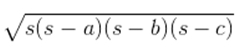Guru

# An umbrella is made by stitching 10 triangular pieces of cloth of two different colours (see Fig.12.16), each piece measuring 20 cm, 50 cm and 50 cm. How much cloth of each colour is required for the umbrella? Q.6

• 0

Please give me the best way for solving the problem of class 9th ncert math of Heron’s Formula chapter of math of class 9th of exercise 12.2  of question no 6 what is the tricky way for solving this question An umbrella is made by stitching 10 triangular pieces of cloth of two different colours (see Fig.12.16), each piece measuring 20 cm, 50 cm and 50 cm. How much cloth of each colour is required for the umbrella?

Share

1. For each triangular piece, The semi perimeter will be

s = (50+50+20)/2 cm = 120/2 cm = 60cm

Using Heron’s formula,

Area of the triangular piece

== √[60(60-50)(60-50)(60-20)] cm2

= √[60×10×10×40] cm2

= 200√6 cm2

∴ The area of all the triangular pieces = 5 × 200√6 cm2 = 1000√6 cm2

• -1